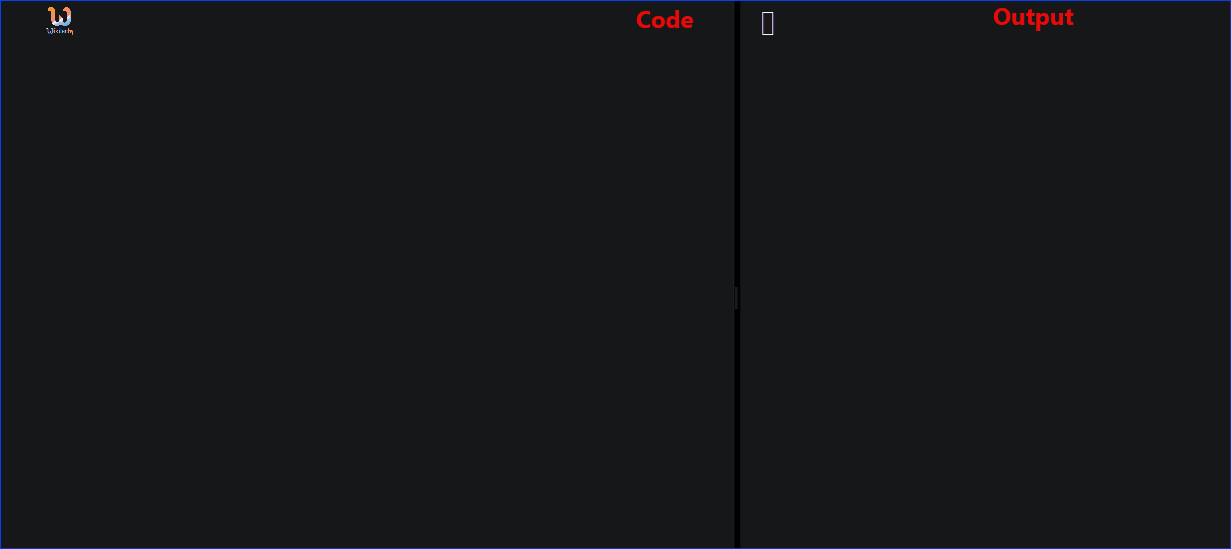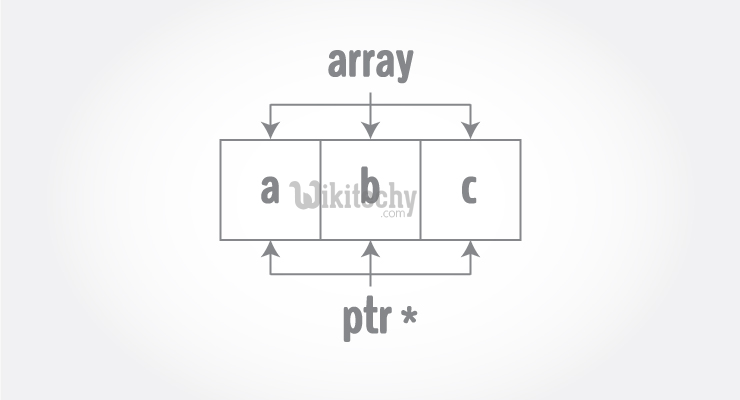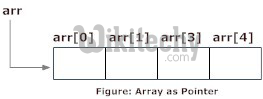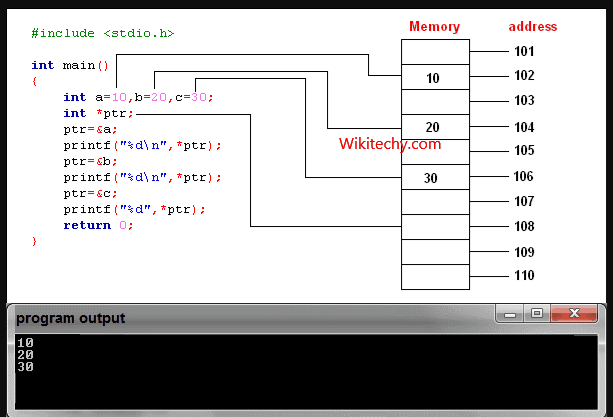# pointer to array | array of pointers | C++ Pointers and Arrays - Learn C++ - C++ Tutorial - C++ programmingLearn c++ - c++ tutorial - pointer-to-array-in-c++ - c++ examples - c++ programs• Pointers are the variables that hold address. Not only can pointers store address of a single variable, it can also store address of cells of an array.
• Consider this example:• Suppose, pointer needs to point to the fourth element of an array, that is, hold address of fourth array element in above case.
• Since ptr points to the third element in the above example, ptr + 1 will point to the fourth element.
• You may think, ptr + 1 gives you the address of next byte to the ptr. But it's not correct.
• This is because pointer ptr is a pointer to an int and size of int is fixed for a operating system (size of int is 4 byte of 64-bit operating system). Hence, the address between ptrand ptr + 1 differs by 4 bytes.
• If pointer ptr was pointer to char then, the address between ptr and ptr + 1 would have differed by 1 byte since size of a character is 1 byte.learn c++ tutorials - pointers in c++ Example

## Reference operator (&) as discussed above gives the address of a variable.

• To get the value stored in the memory address, we use the dereference operator (*).
• For example: If a number variable is stored in the memory address 0x123, and it contains a value 5.
• The reference (&) operator gives the value 0x123, while the dereference (*) operator gives the value 5.
• Note: The (*) sign used in the declaration of C++ pointer is not the dereference pointer. It is just a similar notation that creates a pointer.

## Example 1: C++ Pointers and Arrays

• C++ Program to display address of elements of an array using both array and pointers

## Output

• In the above program, a different pointer ptr is used for displaying the address of array elements arr.
• But, array elements can be accessed using pointer notation by using same array name arr. For example:

## Example 2: Pointer and Arrays

• C++ Program to display address of array elements using pointer notation

## Output

• You know that, pointer ptr holds the address and expression *ptr gives the value stored in the address.
• Similarly, you can get the value stored in the pointer ptr + 1 using *(ptr + 1).
• Consider this code below:
• &ptr is equal to ptr and *ptr is equal to ptr
• &ptr is equal to ptr + 1 and *(ptr + 1) is equal to ptr
• &ptr is equal to ptr + 2 and *(ptr + 2) is equal to ptr
• &ptr[i] is equal to ptr + i and *(ptr + i) is equal to ptr[i]

## Example 3: C++ Pointer and Array

• C++ Program to insert and display data entered by using pointer notation.